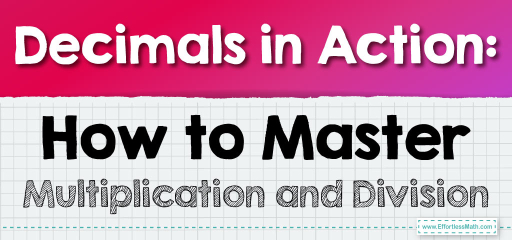# Decimals in Action: How to Master Multiplication and Division

Decimals, being a representation of fractions in base-10, play a crucial role in everyday mathematics. Multiplying and dividing them might seem challenging at first, but with the right approach, it becomes straightforward. Let's dive deep into the world of multiplying and dividing decimals.Multiplying Decimals:

When multiplying decimals, you can initially ignore the decimal points and multiply the numbers as if they were whole numbers. Afterward, count the total number of decimal places in both numbers and place the decimal in the product accordingly.

## Multiplying and Dividing Decimals

### Example 1:

Multiply $$0.4$$ by $$0.3$$.

Solution Process:

Multiply $$4$$ by $$3$$ to get $$12$$. Since there is one decimal place in each number, the product will have two decimal places.

$$0.4 \times 0.3 = 0.12$$.

Dividing Decimals:

When dividing decimals, the goal is to make the divisor a whole number. This is achieved by shifting the decimal point in both the divisor and the dividend.

The Absolute Best Book for 5th Grade Students

### Example 2:

Divide $$0.8$$ by $$0.2$$.

Solution Process:

Shift the decimal in both numbers to make the divisor $$2$$. Now, divide $$8$$ by $$2$$.

$$0.8 \div 0.2 = 4$$.

Multiplying and dividing decimals are essential skills in mathematics. The key is to simplify the process by temporarily converting decimals to whole numbers, performing the operation, and then adjusting for the decimal places. With consistent practice, you’ll find that working with decimals becomes second nature. So, whether you’re calculating discounts, converting units, or solving complex problems, these skills will always come in handy. Dive into the decimal challenges and emerge as a decimal champion!

### Practice Questions:

1. Multiply $$0.5$$ by $$0.6$$.

2. Divide $$0.9$$ by $$0.3$$.

3. Multiply $$0.7$$ by $$0.4$$.

4. Divide $$0.56$$ by $$0.8$$.

5. Multiply $$0.3$$ by $$0.9$$.

A Perfect Book for Grade 5 Math Word Problems!

1. $$0.3$$

2. $$3$$

3. $$0.28$$

4. $$0.7$$

5. $$0.27$$

The Best Math Books for Elementary Students

### What people say about "Decimals in Action: How to Master Multiplication and Division - Effortless Math: We Help Students Learn to LOVE Mathematics"?

No one replied yet.

X
51% OFF

Limited time only!

Save Over 51%

SAVE $15 It was$29.99 now it is \$14.99